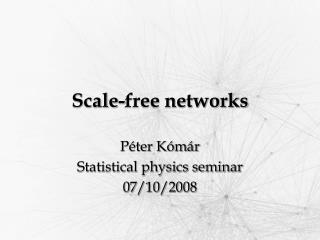Download PresentationScale - free networks

# Scale - free networks

Download Presentation## Scale - free networks

- - - - - - - - - - - - - - - - - - - - - - - - - - - E N D - - - - - - - - - - - - - - - - - - - - - - - - - - -
##### Presentation Transcript

1. Scale-free networks Péter Kómár Statistical physics seminar 07/10/2008

2. Non-regulargraph Elements of graph theory I. • A graph consists: • vertices • edges • Edges can be: • directed/undirected • weighted/non-weighted • self loops • multiple edges

3. Elements of graph theory II. • Degree of a vertex: • the number of edges going in and/or out • Diameter of a graph: • distance between the farthest vertices • Density of a graph: • sparse • dense

4. Networks around us I. • Internet: • routers • cables • WWW: • HTML pages • hyperlinks • Social networks: • people • social relationship

5. Networks around us II. • Transportation systems: • stations / routes • routes / stations • Nervous system: • neurons • axons and dendrites • Biochemical pathways: • chemical substances • reactions

6. Real networks • Properties: • Self-organized structure • Evolution in time (growing and varying) • Large number of vertices • Moderate density • Relatively small diameter (Small World phenomenon) • Highly centralized subnetworks

7. Random networks • Measuring real networks: • Relevant state-parameters • Evolution in time • Creating models: • Analytical formulas • Growing phenomenon • Checking: • ‘Raising’ random networks • Measuring

8. actors www Scale-free property • 1999. A.-L. Barabási, R. Albert • measured the vertex degree distribution → power-law tail: • movie actors: • www: • US power grid: A.-L. Barabási, R. Albert (1999) ‘Emergence of Scaling in Random Networks’, Science Vol. 286

9. Small diameter • 2000. A.-L. Barabási, R. Albert • measured the diameter of a HTML graph • 325 729 documents, 1 469 680 links • found logarithmic dependence: • ‘small world’ A.-L. Barabási, R. Albert, H. Jeong (2000) ‘Scale-free characteristics of random networks: the topology of the wold-wide web’, Physica A, Vol. 281 p. 69-77

10. pER = 6∙10-4 N=104 10-3 1.5∙10-3 Erdős-Rényi graph (ER) (1960. P. Erdős, A. Rényi) • Construction: • N vertices • probability of each edge: pER • Properties: • pER ≥ 1/N → → Asympt. connected • degree distribution: Poisson (short tail) • not centralized • small diameter A.-L. Barabási, R. Albert, H. Jeong (1999) ‘Mean-field theory for scale-free random networks’, Physica A, Vol. 272 p.173-187

11. ER graph example

12. Small World graph (WS) (1998. D.J. Watts, S.H. Strogatz) • Construction: • N vertices in sequence • 1st and 2nd neighbor edges • rewiring probability: pWS • Properties: • pWS = 0 → clustered, • 0 < pWS < 0.01 → clustered → small-world propery • pWS = 1 → not clustered, A.-L. Barabási, R. Albert, H. Jeong (1999) ‘Mean-field theory for scale-free random networks’, Physica A, Vol. 272 p.173-187

13. WS graph example

14. ER graph - WS graph WS ER

15. m0= 3 m = 2 Barabási-Albert graph (BA) • New aspects: • Continuous growing • Preferential attachment • Construction: • m0 initial vertices • in every step: +1 vertex with m edges • P(edge to vertex i) ~ degree of i A.-L. Barabási, R. Albert, H. Jeong (1999) ‘Mean-field theory for scale-free random networks’, Physica A, Vol. 272 p.173-187

16. 7 5 3 1 = m0 =m N = 300 000 Barabási-Albert graph II. • Properties: • Power-law distribution of degrees: • Stationary scale-free state • Very high clustering • Small diameter A.-L. Barabási, R. Albert, H. Jeong (1999) ‘Mean-field theory for scale-free random networks’, Physica A, Vol. 272 p.173-187

17. BA graph example

18. ER graph – BA graph

19. ki(t) ~ t 1/2 t ti Mean-field approximation I. • Time dependence of ki (continuous): • solution: probability of anedge to ith vertex time of occurrence of the ith vertex A.-L. Barabási, R. Albert, H. Jeong (1999) ‘Mean-field theory for scale-free random networks’, Physica A, Vol. 272 p.173-187

20. Mean-field approximation II. • Distribution of degrees: • Distribution of ti : • Probability density: A.-L. Barabási, R. Albert, H. Jeong (1999) ‘Mean-field theory for scale-free random networks’, Physica A, Vol. 272 p.173-187

21. p(k) exponential scale-free k Without preferential attachment • Uniform growth: • Exponential degree distribution: A.-L. Barabási, R. Albert, H. Jeong (1999) ‘Mean-field theory for scale-free random networks’, Physica A, Vol. 272 p.173-187

22. t = N N=10 000 5N 40N Without growth • Construction: • Constant # of vertices • + new edges with preferential attachment • Properties: • At early stages → power-law scaling • After t ≈ N2 steps → dense graph A.-L. Barabási, R. Albert, H. Jeong (1999) ‘Mean-field theory for scale-free random networks’, Physica A, Vol. 272 p.173-187

23. Conclusion • Power-law = Growth + Pref. Attach. • Varieties • Non-linear attachment probability: → affects the power-law scaling • Parallel adding of new edges → • Continuously adding edges (eg. actors) → may result complete graph • Continuous reconnecting (preferentially) → may result ripened state A.-L. Barabási, R. Albert, H. Jeong (1999) ‘Mean-field theory for scale-free random networks’, Physica A, Vol. 272 p.173-187

24. Network research today Centrality Adjacency matrix Spectral density Attack tolerance A.-L. Barabási, R. Albert,‘Statistical Mechanics of Complex Networks’, arXiv:cond-mat/0106096 v1 6 Jun 2001

25. Thank you for the attention!

26. ER – WS – BA